PRINTABLE FOR KIDS

XII (12) HSC

XI (11) FYJC
X (10) SSC

### Heat Class 10th Science And Technology Part 1 MHB Solution

Exercise

###### Exercise
Question 1.

Fill in the blanks and rewrite the sentence.

a. The amount of water vapor in air is determined in terms of its …………

b. If objects of equal masses are given equal heat, their final temperature will be different. This is due to difference in their ……………...

c. During transformation of liquid phase to solid phase, the latent heat is ………….

(a) The amount of water vapor in air is determined in terms of its temperature.

Explanation:

The amount of vapor needed to saturate the air depends on temperature of the air.

⇒ If the temperature is low, less vapor is needed to saturate the air.

⇒ If the temperature is high, additional vapor will condense.

(b) If objects of equal masses are given equal heat, their final temperature will be different. This is due to difference in their specific heat capacity.

Explanation:

The amount of heat energy required to raise the temperature of a unit mass of an object by 1°C is called the specific heat capacity of that object.

(c) During transformation of liquid phase to solid phase, the latent heat is absorbed.

Explanation:

During transition of solid phase to liquid phase, the objects absorbs heat energy. This heat energy is used for breaking the bonds between the atoms or molecules in the solid and transform it into solid phase.

Question 2.

Observe the following graph. Considering the change in volume of water as its temperature is raised from 0oC, discuss the difference in the behaviour of water and other

substances. What is this behavior of water called?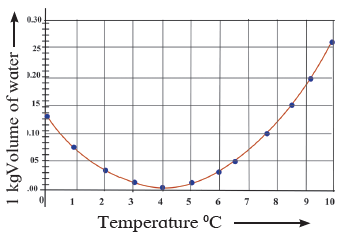The given figure is the graph between the volume and temperature of water.

(i) If the temperature is raised from 0°C, this means heating takes place.

(ii) It has been observed that on heating, it contracts instead of expanding.

(iii) At 4° C, due to contraction, volume decreases.

(iv) If the water is heated further, it expands and its volume increases.

(v) The behaviour of water between its temperature from 4° C, to 0° C, is called anomalous behaviour of water.

Question 3.

What is meant by specific heat capacity? How will you prove experimentally that different substances have different specific heat capacities?

The amount of heat energy required to raise the temperature of a unit mass of an object by 1° C is called the specific heat capacity of that object.

To prove experimentally: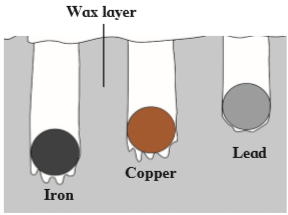(i) Take three spheres of iron, copper and lead of equal masses.

(ii) Now put all the three spheres in boiling water in the beaker for some time.

(iii) Now, take them all out of the water, we will notice that all the spheres will be at temperature 100° C.

(iv) Now, put them immediately on the thick slab of wax.

(v) Note the depth of each sphere into the wax.

(vi) The sphere which has absorbed more heat from water will give more heat to wax.

(vii) More wax will thus melt, and deeper the sphere will go in the wax.

(viii) We will notice, iron goes deepest, lead sphere goes the least and copper sphere goes to intermediate depth.

(ix) This shows that for equal rise in temperature, the three spheres have absorbed different amounts of heat.

(x) This means that the property which determines the amount of heat absorbed by a sphere is different for the three spheres.

(xi) This property is called the specific heat capacity.

Question 4.

While deciding the unit for heat, which temperatures interval is chosen? Why?

While defining the unit for heat, the temperature range between 14°C to 15.5°C is chosen.

If we take different temperature range than 14°C to 15.5°C, the amount of heat required will be slightly different. It is, therefore, essential to define a specific temperature range while.

defining the unit of heat.

Question 5.

Explain the following temperature vs time graph.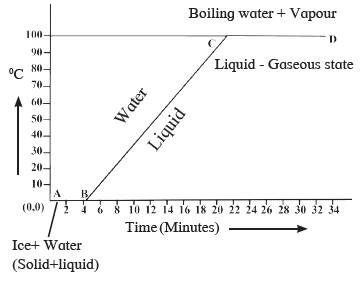Temperature vs time graph:

i. In this graph, line AB shows conversion of ice into water at constant temperature. Thus, AB represents: ice + water

ii. The temperature remains constant during this transition. This transition temperature at which ice changes to water is called melting point of ice.

iii. Line BC shows the rise in temperature of water from 0° to 100° C. This line represents: solid stateliquid state.

iv. Thereafter, heat energy is supplied to water. The heat energy absorbed by water which is used to break the bonds of liquid molecules and convert the liquid into gaseous state.

v. Point C represents: liquid stategaseous state

vi. The constant temperature at which the liquid transforms into gaseous state is called the boiling point of the liquid.

vii. In last, the water completely changes to vapor.

viii. This whole process is called latent heat of vaporization.

Question 6.

Explain the following:

What is the role of anomalous behaviour of water in preserving aquatic life in regions of cold climate?

The behavior of water between its temperature from 0° C to 4°C is called anomalous behaviour of water.

Role of anomalous behaviour of water in preserving aquatic life:

(i) In winter, as the temperature of the atmosphere goes down due to which the water of the surface also cools down.

(ii) As a result, the temperature of whole water body decreases to 4°C.

(iii) Now, as water on the surface at 4° C cool down further, its volume increases and density also increases.

(iv) On reaching the temperature at 0° C, water transforms into ice.

(v) The process of cooling becomes slow due to the low thermal conductivity of ice (ice bad conductor of heat).

(vi) Thus, a temperature of 4° C is always maintained.

(vii) In normal conditions, the temperature goes on increasing.

(viii) This whole process preserves the aquatic life even in cold regions.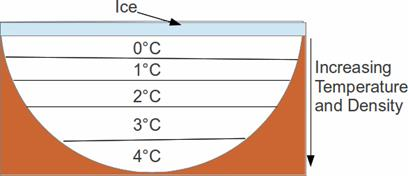Question 7.

Explain the following:

How can you relate the formation of water droplets on the outer surface of a bottle taken out of refrigerator with formation of dew?

The water vapor of the air depends upon the specific temperature.

Dew point: An unsaturated air at a certain temperature is taken and its temperature is decreased, a temperature is reached at which the air becomes saturated with vapor. This temperature is called the dew point temperature.

Saturated with vapor: If the amount of air exceeds this limit, the excess vapor converts into water droplets. When the air contains maximum possible water vapor, it is said to be saturated with vapor at that temperature.

Now, if we take a water bottle out of a refrigerator, we will observe tiny water droplets on it:

(i) This means, due to decrease in temperature, the air cools.

(ii) It becomes saturated with water vapor.

(iii) As a result, the excess water vapor gets converted into tiny droplets.

(iv) The dew-point temperature is decided by the amount of vapor in the air.

Question 8.

Explain the following:

In cold regions in winter, the rocks crack due to anomalous expansion of water.

Anomalous expansion: Water contracts when heated from 0° to 4°C (instead of expanding) and water expands when cooled from 4°C to 0° This expansion of water from 4°C to 0° C is called anomalous expansion of water.

(i) In colder seasons, the temperature drops to 4°C.This means water starts to expand.

(ii) As there is no place for the water to expand inside the rocks, it exerts a high pressure on the rocks.

(iii) As a result, the rocks break.

Question 9.

What is meant by latent heat? How will the state of matter transform if latent heat is given off?

Latent heat: The amount of heat energy required by any substance to convert from one state to another state is called latent heat. There are two types of latent heat:

1. Latent heat of fusion

2. Latent heat of vaporization

⇒ When latent heat of fusion is applied, the substance changes from solid phase to liquid phase. This means the substance absorbs heat energy which is used to break the bonds of the solid atoms and transform it into liquid phase.

⇒ When latent heat of vaporization was applied, the water in liquid state transforms into gaseous state. This means the water absorbs heat energy which is used to break the bonds of the liquid molecules and convert the liquid into gaseous state.

Question 10.

Which principle is used to measure the specific heat capacity of a substance?

Principle of heat capacity:

⇒ If heat is exchanged between hot and cold object, the temperature of the cold object increases (energy gain) and the temperature of the hot object decreases (energy loss).

⇒ Until the objects attain the same value, the temperature continues to change.

⇒ If the system of both the objects is isolated and close in a heat resistant box, then no energy can flow from inside the box or come into the box.

⇒ In this condition, we get the following principle:

Heat energy lost by the hot object = Heat energy gained by the cold object.

Question 11.

Explain the role of latent heat in the change of state of a substance?

The role of latent heat in the change of state of a substance are:

⇒ Latent heat of fusion: The latent heat of fusion helps to transform any substance from solid state to liquid state by absorbing heat energy at constant temperature during transformation.

⇒ Latent heat of vaporization: The latent heat of vaporization helps to transform any liquid substance from liquid state to gaseous state by absorbing heat energy at constant temperature during transformation.

Question 12.

On what basis and how will you determine whether air is saturated with vapor or not?

To determine whether air is saturated with vapor or not, it is based on the temperature of the air:

i. If air temperature is low, the air is saturated with less vapor.

ii. If the air temperature is high, the air is saturated with additional vapor.

iii. If the vapor contained in air is less that the maximum limit, then the air is said to be unsaturated.

Question 13.

Read the following paragraph and answer the questions.

If heat is exchanged between a hot and cold object, the temperature of the cold object goes on increasing due to gain of energy and the temperature of the hot object goes on decreasing due to loss of energy.

The change in temperature continues till the temperatures of both the objects attain the same value. In this process, the cold object gains heat energy and the hot object loses heat energy. If the system of both the objects is isolated from the environment by keeping it inside a heat resistant box (meaning that the energy exchange takes place between the two objects only), then no energy can flow from inside the box or come into the box.

i. Heat is transferred from where to where?

ii. Which principle do we learn about from this process?

iii. How will you state the principle briefly?

iv. Which property of the substance is measured using this principle?

i. Heat energy exchange takes place between the two objects only.

Because is system is isolated (has no interaction with surroundings) so no energy can flow from inside the box or come into the box.

ii. The principal which we learnt from the process is:

Heat energy lost by the hot object = Heat energy gained by the cold object.

iii. In the principle:

⇒ The cold objects gain heat energy and the hot object loses heat energy.

⇒ If the system become isolated (by using a heat resistant box), then the heat energy takes place between the two objects only.

⇒ As a result, no energy can flow from inside the box or come into the box.

⇒ In this situation, we get the following principle

Heat energy lost by the hot object = Heat energy gained by the cold object.

iv. The specific heat of the substance is measured using this principle:

⇒ The specific heat of the substance is measured by mixing method.

⇒ For this, calorimeter is used.

Question 14.

Solve the following problems:

Equal heat is given to two objects A and B of mass 1 g. Temperature of A increases by 3oC and B by 5oC. Which object has more specific heat? And by what factor?

Specific heat is the amount of heat per unit mass required to raise the temperature by one degree Celsius.

It is given by the formula:

Q = mcΔT

Or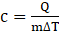Where, Q is the amount of heat added.

T is the temperature.

“c” is the specific heat

“m” is the mass

Now, let say, two objects A and B.

Then, the ratio of the specific heats of two objects is given as: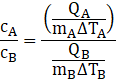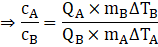As per the question, equal heat is given to two objects A and B of mass 1 g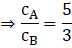Or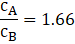Clearly, it shows that the specific heat of A is greater than B with a factor 1.66.

Question 15.

Solve the following problems:
Liquid ammonia is used in an ice factory for making ice from water. If the water at 20oC is to be converted into 2 kg ice at 0oC, how many grams of ammonia is to be evaporated?

(Given: The latent heat of vaporization of ammonia= 341 cal/g)

Given: Latent heat of vapourisation of Ammonia =341 cal/g
Mass of ammonia be= M
Mass of water =2000g
Temperature change, ΔT=20oC-0oC=20oC

latent heat is the heat required by the ammonia for the liquid ammonia to ammonia vapours.

Amount of heat energy released in colling 2 Kg water from 20oC to 0oC
QW=MCΔT

Where
M is the mass of water
C is specific at constant volume
ΔT is the temperature difference

Putting the values in the above formula, we get
Qw=2000×1×20=40000 cal

Amount of heat energy released during the conversion of 2kg of water at 0oC to ice at 0oC
Qi =M×L

Where
M is the mass of the water
L is the latent heat of conversion of water to ice
Putting the values in the above equation, we get
Qi=2000×80=160000 cal

The heat gained by ice due presence of ammonia will be:

Q = m× L=Qi +Qw

Where
m is the mass of the ammonia
L is the latent heat of the ammonia
On solving, we get
m× L=160000+40000=200000 cal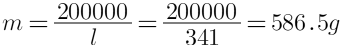Thus, the process of conversion of 2kg of water to ice will require evaporation of 586.5 g of ammonia

Question 16.

Solve the following problems:
A thermally insulated pot has 150 g ice at temperature 0oC. How much steam of 100oC has to be mixed to it, so that water of temperature 50oC will be obtained? (Given: latent heat of melting of ice = 80 cal/g, latent heat of vaporization of water = 540 cal/g, the specific heat of water = 1 cal/g °C)

Answer: 1 g of ice needs 80 cal to become water at 0 C.
1 g of water needs 50 cal to rise to 50 C from 0 C.
So total the heat needed is 150×80 + 150×50 = 19500 cal.
1 g of steam will supply 540 c to become water at 100 C.
1 g of water at 100 C will supply 50 cal to become water at 50 C.
Hence x gram of steam at 100 C will supply 590 cal while cooling to 50 C.
Hence 19500 = 590 x.
Solving, x = 33.05 gram.

Question 17.

Solve the following problems:

A calorimeter has mass 100 g and specific heat 0.1 kcal/ kg°C. It contains 250 gm of liquid at 30°C having a specific heat of 0.4 kcal/kg°C. If we drop a piece of ice of mass 10g at 0°C, What will be the temperature of the mixture?

we know that 1g = 7.716 Cal
Now,
100 g = 771.6 cal 771.6/1000 = 0.7716 kCal
250 g = 1929 cal = 1929/1000 = 1.929 kCal
10 g = 77.16 cal = 77.16/1000 = 0.07716 kCal

heat of fusion in ice(Latent) = 80 k Cal / Kg
We know the formula:-

Heat lost in the process = (M(mass)× C(heat capacity) × ΔT(change in temperature)) -------→(i)

As we know, the ice dropped there, that's the heat loss.

Therefore the Change in temperature = (30 - T) ) ×Heat heat gained by ice = heat lost by the liquid & calorimeter

Substituting this values in the equation (i)

⇒ the final temperature of the calorimeter + final temperature of liquid = Heat loss due to the ice at 00c

⇒ (30 - T) × 0.7716 + (0.1+ (30 - T) ) × { 1.926 × 0.4 } = {0.07716 ×80 } + { T× 0.07716 × 1}

⇒ { 2.3148 - 0.07716) × T + 23.112 -0.7704) × T } = { 6.1728 + 0.07716 ) ×T }

⇒ {2.3148 +23.112 - 6.1728} = {0.07716 + 0.7704 + 0.07716 }

⇒ 0.92472 T = 19.254

⇒T=20.80c

## PDF FILE TO YOUR EMAIL IMMEDIATELY PURCHASE NOTES & PAPER SOLUTION. @ Rs. 50/- each (GST extra)

SUBJECTS

HINDI ENTIRE PAPER SOLUTION

MARATHI PAPER SOLUTION
SSC MATHS I PAPER SOLUTION
SSC MATHS II PAPER SOLUTION
SSC SCIENCE I PAPER SOLUTION
SSC SCIENCE II PAPER SOLUTION
SSC ENGLISH PAPER SOLUTION
SSC & HSC ENGLISH WRITING SKILL
HSC ACCOUNTS NOTES
HSC OCM NOTES
HSC ECONOMICS NOTES
HSC SECRETARIAL PRACTICE NOTES

2019 Board Paper Solution

HSC ENGLISH SET A 2019 21st February, 2019

HSC ENGLISH SET B 2019 21st February, 2019

HSC ENGLISH SET C 2019 21st February, 2019

HSC ENGLISH SET D 2019 21st February, 2019

SECRETARIAL PRACTICE (S.P) 2019 25th February, 2019

HSC XII PHYSICS 2019 25th February, 2019

CHEMISTRY XII HSC SOLUTION 27th, February, 2019

OCM PAPER SOLUTION 2019 27th, February, 2019

HSC MATHS PAPER SOLUTION COMMERCE, 2nd March, 2019

HSC MATHS PAPER SOLUTION SCIENCE 2nd, March, 2019

SSC ENGLISH STD 10 5TH MARCH, 2019.

HSC XII ACCOUNTS 2019 6th March, 2019

HSC XII BIOLOGY 2019 6TH March, 2019

HSC XII ECONOMICS 9Th March 2019

SSC Maths I March 2019 Solution 10th Standard11th, March, 2019

SSC MATHS II MARCH 2019 SOLUTION 10TH STD.13th March, 2019

SSC SCIENCE I MARCH 2019 SOLUTION 10TH STD. 15th March, 2019.

SSC SCIENCE II MARCH 2019 SOLUTION 10TH STD. 18th March, 2019.

SSC SOCIAL SCIENCE I MARCH 2019 SOLUTION20th March, 2019

SSC SOCIAL SCIENCE II MARCH 2019 SOLUTION, 22nd March, 2019

XII CBSE - BOARD - MARCH - 2019 ENGLISH - QP + SOLUTIONS, 2nd March, 2019

HSC Maharashtra Board Papers 2020

(Std 12th English Medium)

HSC ECONOMICS MARCH 2020

HSC OCM MARCH 2020

HSC ACCOUNTS MARCH 2020

HSC S.P. MARCH 2020

HSC ENGLISH MARCH 2020

HSC HINDI MARCH 2020

HSC MARATHI MARCH 2020

HSC MATHS MARCH 2020

SSC Maharashtra Board Papers 2020

(Std 10th English Medium)

English MARCH 2020

HindI MARCH 2020

Hindi (Composite) MARCH 2020

Marathi MARCH 2020

Mathematics (Paper 1) MARCH 2020

Mathematics (Paper 2) MARCH 2020

Sanskrit MARCH 2020

Important-formula

THANKS